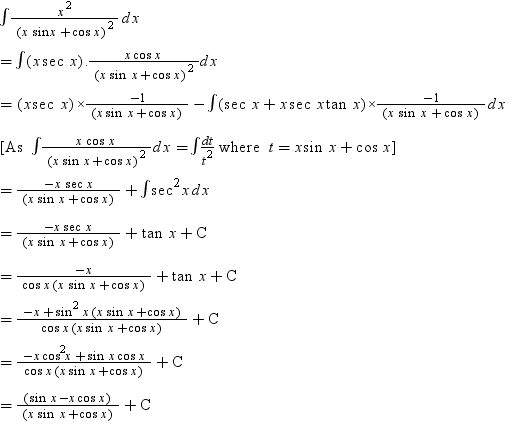# Integration by parts for definite integral

$$\int_{0}^{\frac{\pi}{2}} \frac{x^2}{(x\sin\ x+\cos\ x)^2}=\left[\frac{-x\sec\ x}{(x\sin\ x+\cos x)^2}\right]_0^\frac{\pi}{2}+\int_{0}^{\frac{\pi}{2}} \sec^2 x dx$$

Is this a correct application of integration by parts for definite integral?

Clearly, $$\int_{0}^{\frac{\pi}{2}} \sec^2 x\ dx$$ does not exist.

The right hand side is in the form $$\infty -\infty$$.

If this is incorrect, how should integration by parts be applied here?

The indefinite integral is shown as below. By substituting the upper and lower limits, the answer of the definite integral should be $$\frac{2}{\pi}$$.• In general, it is possible to pick such an unfortunate combination of functions $u, ~dv$ for integration by parts, that $uv |_a^b$ doesn't exist. You have to be careful in what you pick – Yuriy S Oct 14 '18 at 9:34
• @YuriyS Then, what is the correct way to apply integration by parts here? – Stupid Girl Oct 14 '18 at 10:07
• @YuriyS The expression on top is just the 5th line of the indefinite integral with upper and lower limits. – Stupid Girl Oct 14 '18 at 10:40
• @YuriyS Sorry for a typo previously, it was supposed to be "u=x sec x, v=-1/(x sin x+cos x), u is undefined when x=pi/2". This is the same choice of u and v as the indefinite integral. – Stupid Girl Oct 14 '18 at 10:43
• I see. Clearly, as you notice, for these particular limits this choice does lead to the undetermined expression $\infty-\infty$, which, however can be solved by taking indefinite integral in the second term first, and only applying limits to the final expression. It is allowed. Otherwise, you'd have to pick different $u,dv$ – Yuriy S Oct 14 '18 at 10:55